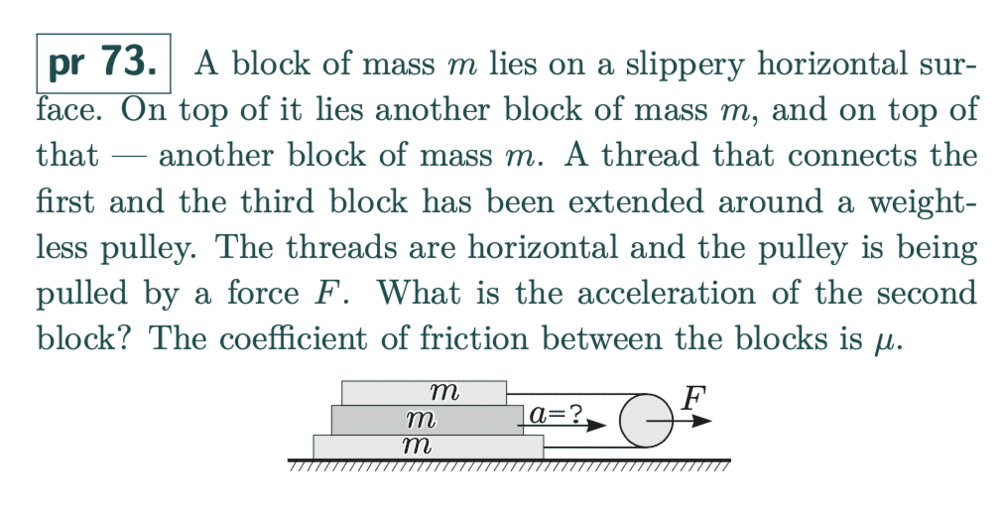# If I pull a pulley, what is the tension in the string around it?

• phantomvommand
Since the string exerts a force of F/2 on the pulley, the pulley experiences a net force of (F-F/2) or F/4 acting on it.

#### phantomvommand

Homework Statement
Relevant Equations
F = maI assumed that each of the masses attached to the string would be pulled with a force F, instead of F/2, which appears to be the correct value. Why is it F/2? I suspect the fact that the pulley is "weightless" has something to do with the F/2 value. What is it?

Thank you.

phantomvommand said:
Why is it F/2? I suspect the fact that the pulley is "weightless" has something to do with the F/2 value.
Yes indeed. Since the pulley is massless, there cannot be a net force on it -- if there was, its acceleration would be infinite.

Doc Al said:
Yes indeed. Since the pulley is massless, there cannot be a net force on it -- if there was, its acceleration would be infinite.
thanks for this. What is not clear to me is how the F/2 force in each branch of the string acts to negate the F force on the pulley. At the "bend" of the string, wouldn't the forces there just be F/2 in 2 opposite directions, both of which are perpendicular to the direction of F on the pulley? How does the pulley experience the opposing force of F?

Perhaps because I'm lazy, but I just imagine a "box" around the pulley with the two strings pulling one way and the force F pulling the other way. That way I can avoid worrying about the details of the interaction between the pulley and the string as it goes around the bend. (Note that there will be "normal" forces acting on the string as it bends around the pulley.)

•phantomvommand
Summation of forces and moments about the axis of that pulley are both zero, as the thing is not rotating or falling apart.

In order to be a balance of x-axis forces acting on the pulley, T1+T2=F.
For ideal conditions of no mass and no friction in the axis of the pulley, T1=T2.